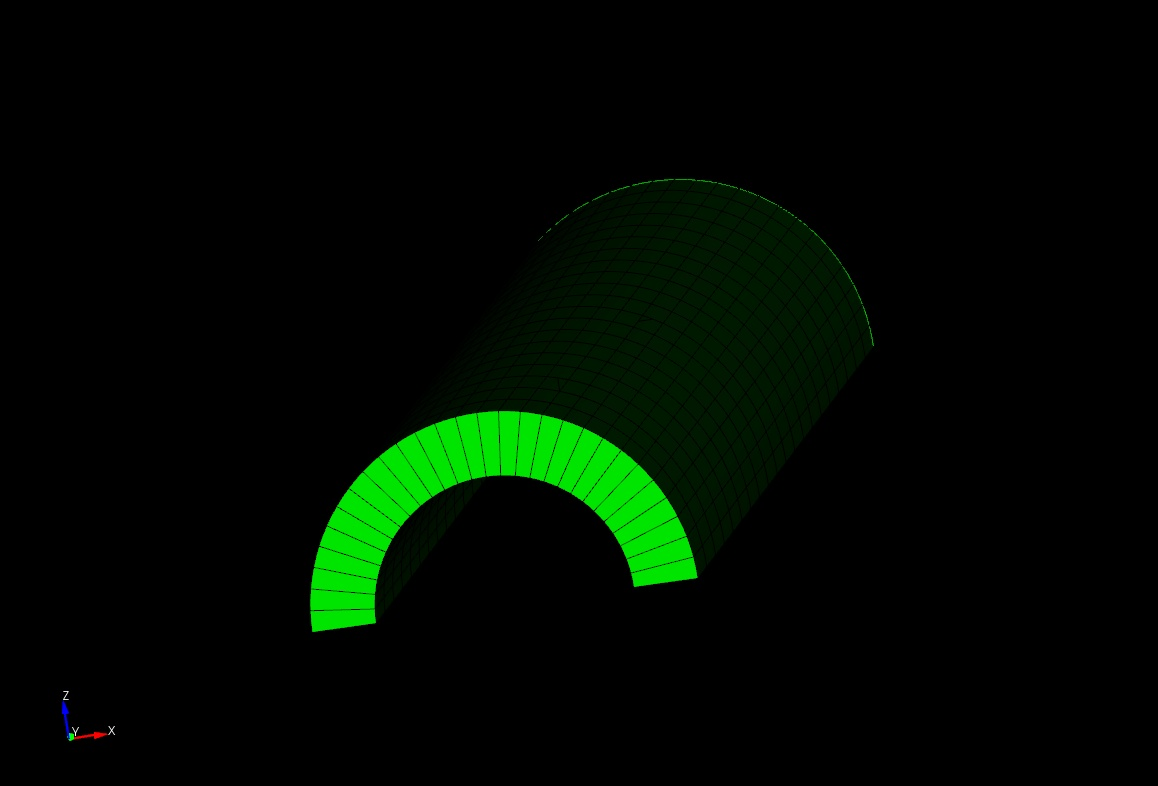# Creation of cylindrical thin shell structure

Hello,

I am new to CUBIT and would like to create a thin shell structure. I wrote a python script for the same and it is as follows,

#!python
cubit.cmd(‘reset’)

cubit.cmd(‘create vertex -3.20 0 0’)
cubit.cmd(‘create vertex 0 0 0’)
cubit.cmd(‘create vertex 3.20 0 0’)

cubit.cmd(‘create vertex -2.93 0 0’)
cubit.cmd(‘create vertex 2.93 0 0’)

cubit.cmd(‘create curve arc center vertex 2 4 5 radius 2.93 normal y’)
cubit.cmd(‘create curve arc center vertex 2 1 3 radius 3.20 normal y’)

cubit.cmd(‘create curve 4 1’)
cubit.cmd(‘create curve 3 5’)

cubit.cmd(‘create surface 1 2 3 4’)
cubit.cmd(‘curve 4,3 interval 1’)
cubit.cmd(‘surface 1 scheme map’)
cubit.cmd(‘mesh surface 1’)

cubit.cmd('sweep surface 1 perpendicular distance 40 ')

cubit.cmd(‘curve 8,12 interval 1’)
cubit.cmd(‘surface 6 scheme map’)
cubit.cmd(‘mesh surface 6’)

cubit.cmd(‘curve all with length 40 interval 20’)
cubit.cmd(‘block 1 volume 1’)
cubit.cmd(‘block 1 element type HEX27’)
cubit.cmd(‘mesh volume 1’)

However CUBIT 13.2 is not accepting floating point numbers as vertices (i.e. in lines 4,6,8 and 9, the vertices -3.20, 3.20, 2.93 and -2.93 are converted to -3,3,2 and -2). Hence my shell thickness becomes 1 unit which is wrong. I would like to know if my method is the right way to create a thin shell structure or if there is another way?

Thank you!I don’t see the same problem in Trelis or Cubit 15.3 (thickness is .27). We don’t support Cubit 13.2 anymore (released 2012). You might contact Sales to update your license (sales@csimsoft.com).

Thank you for your reply! I guess its a problem with my version of CUBIT.